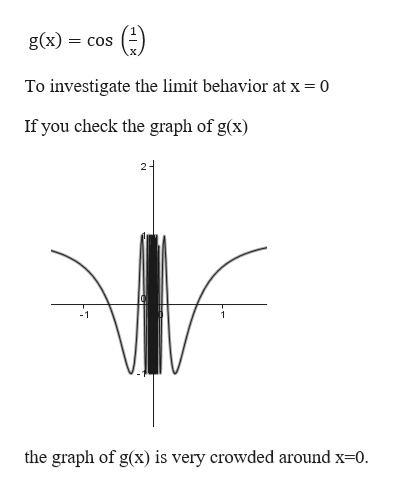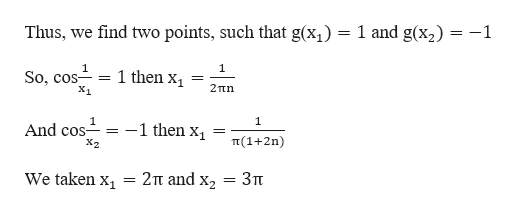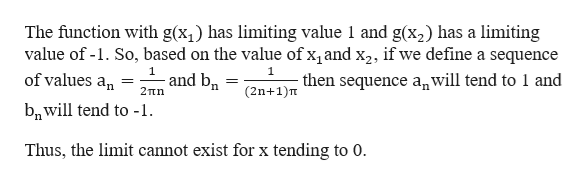Consider the function g(x) = cos(1/x). We will investigate the limit behavior at x = 0.c) If n is an arbitrary positive integer, find points x1 and x2 (int terms of n) in the interval(-1/n, 1/n( such that g(x2) = 1 and g(x2) = -1.d) Explain (in a brief paragraph) why (c) implies that g does not have a limit at x = 0.  e) Where is g continuous? Justify your answer. You may use facts from the textbook in Section 2.2 - 2.5.

Question

Consider the function g(x) = cos(1/x). We will investigate the limit behavior at x = 0.

c) If n is an arbitrary positive integer, find points x1 and x2 (int terms of n) in the interval(-1/n, 1/n( such that g(x2) = 1 and g(x2) = -1.

d) Explain (in a brief paragraph) why (c) implies that g does not have a limit at x = 0.

e) Where is g continuous? Justify your answer. You may use facts from the textbook in Section 2.2 - 2.5.

Step 1

Given function,help_outlineImage Transcriptioncloseg(x) = COS To investigate the limit behavior at x = 0 If you check the graph of g(x) 2+ -1 the graph of g(x) is very crowded around x=0 fullscreen
Step 2

The graph of g(x) is very crowded around x = 0.help_outlineImage Transcriptionclose1 and g(x2) -1 Thus, we find two points, such that g(x 1 So, cos X1 1 1 then x _= 2Tn 1 -1 then X1 And cos- = X2 T(1+2n) We taken x 2Tt and x2 3Tt fullscreen
Step 3

d) Now, to check the ...help_outlineImage TranscriptioncloseThe function with g(x has limiting value 1 and g(x2) has a limiting value of -1. So, based on the value of x, and x2, if we define a sequence 1 1 and b of values an (2n+1)Ten sequence anwill tend to 1 and 2πη bnwill tend to -1 Thus, the limit cannot exist for x tending to 0. fullscreen

Want to see the full answer?

See Solution

Want to see this answer and more?

Our solutions are written by experts, many with advanced degrees, and available 24/7

See Solution
Tagged in

Limits